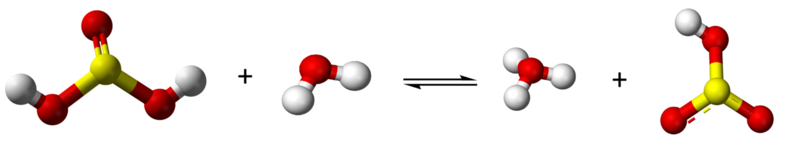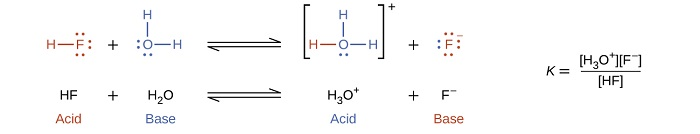# 16.1: Brønsted-Lowry Concept of Acids and Bases

## Introduction

Acids and bases are common substances and the concept of acids and bases have evolved as science progressed. There are three definitions that you will be required to know by the end of this chapter, the Arrhenius, Brønsted-Lowry and Lewis. In general chemistry 1, Section 3.5 you learned how to balance Acid Base Reactions and may want to review that section. There are 4 videos for balancing:

1. Strong Acid and Strong Base
2. Strong Acid and Weak Base
3. Strong Base and Weak Acid
4. Weak Acid and Weak BaseIn this section we will take a deeper look into the nature of acids and bases

### Autoionization of water

Neutral water does not consist of pure H2O, as sometimes when two water molecules collide a proton is transferred from one molecule to another.

$\ce{H_2O} \left( l \right) + \ce{H_2O} \left( l \right) \rightarrow \ce{H_3O^+} \left( aq \right) + \ce{OH^-} \left( aq \right)$

Note, when this happens, the number of positive charged hydronium ions equals the number of negatively charged hydroxide ions, and so even though there are charged particles, the water is still neutral.

Video$$\PageIndex{2}$$ (https://youtu.be/iqINYjHyimY): 10 Youtube uploaded by akumich showing the formation of hydronium ions and hydroxide ions as protons are transferred between water molecules.

The above animation and the longer video it links to does a good job of showing the transient nature of the actual hydronium or hydroxide ions in neutral water. That is, as soon as a hydroxide is formed, its negative net charge will grab a proton from another water molecule to become neutral (but form another hydroxide), and in a similar manner each hydronium will give its extra proton to another water molecule, becoming neutral while forming another hydronium. And of course the hydronium and hydroxide ions will react with each other to form neutral water. It also needs to be emphasized that the concentration of hydronium and hydroxide is 0.0000001 M (10-7M) in pure water, which is why most collisions are with other molecules.

### Arrhenius Concept of Acids and Bases

The Arrhenius Definition is based on the autoionization of water and is limited because it can only describe acids and bases in aqueous environments. Arrhenius defined an acid to be a compound that dissolves in water to make hydronium ions and a base as a compound that dissolves in water to yield hydroxide anions.

#### Arrhenius Acid

An Arrhenius Acid is something that gives a proton to water. So for an Arrhenius Acid:

$H_{3}O^{+}(aq)>OH^{-}$

Note, the solution is still neutral because whatever gave the proton to water became negative. HCl acts as an Arrhenius acid when dissolved in water, and every time an HCl molecule gives a proton to a water molecule it not only forms hydronium but also chloride

$\underset{an\: Arrhenius\: acid}{HCl_{(g)}} \xrightarrow {H_3 O_{(l)}} H^+_{(aq)} + Cl^-_{(aq)}$

#### Arrhenius Base

An Arrhenius base is something that forms hydroxide when added to water. So for an Arrhenius Base:

$H_{3}O^{+}(aq)<OH^{-}$

$\underset{an\: Arrhenius\: base}{NaOH_{(s)}} \xrightarrow {H_2O_{(l)}} Na^+_{(aq)} + OH^-_{(aq)}$

NaOH is an Arrhenius Base as dissolving it in water produces hydroxide ions.

Note, there is another type of base, which is typified by ammonia and the amines, which have a nitrogen with a lone pair of electrons. These grab a proton from neutral water forming hydroxide

${NH_3 (g)} + {H_2 O(l)} \rightleftharpoons {NH_4^+ (aq)} + {OH^- (aq)}$

## Bronsted Concept of Acids and Bases

An Arrhenius Acid is something that donates a proton to water, and Bronsted-Lowry Concept extends this to any substance, where an acid is a proton donor and a base is a proton acceptor.

• Acid = Proton Donor
• Base=Proton Acceptor

Consider the reactions of ammonia with water and HCl. In the first reaction ammonia is both an Arrhenius and a Bronsted base, in the second it is only a Bronsted base.

$\underset{both\: Arrhenius\: and \\ \: Bronsted\: base}{NH_3 (g)} + {H_2 O(l)} \rightleftharpoons {NH_4^+ (aq)} + {OH^- (aq)}$

$\underset{only\: a\: Bronsted\: base} {NH_3 (g)} + {HCl(aq)} \rightleftharpoons {NH_4^+ (aq)} + {Cl^- (aq)}$

So Arrhenius acids are a subset of Bronsted acids, where water is the proton acceptor.

### Conjugate Acid-Base Pairs

Note when molecule "A" gives a proton to molecule "B", molecule molecule "A" acted as an acid and molecule "B" acted as a base.  After this reaction, lets call molecule "A" as "a" to indicate that it is minus a proton, and "B" as "b", to indicate that it has received a proton.  Well, now "b" is a base and has an extra proton it can give away (becoming "B") and "a" is a base, which can accept a proton and become "A".  So "a" and "b" are the conjugate base and conjugate acid of the acid "A' and base"B".  This is demonstrated in figure $$\PageIndex{2}$$.Figure $$\PageIndex{2}$$: Acid/base conjugate pair relationship

From figure 16.1.2 you can see that hydronium is the conjugate acid of water, and chloride is the conjugate base of HCl. This is important as it can be used to explain the acid/base behavior of salt solutions.

### Polyprotic Acids and Bases

An acid that can donate more than one proton is a polyprotic acid, and a base that can accept more than one proton is a polyprotic baseFigure$$\PageIndex{3}$$: Note, not all protons are acidic. In the case of oxyacids, where oxygen is attached to a non-metal, only the protons attached to the oxygen are acidic. This is why H3PO3 and H3PO2 are diprotic and monoprotic respectively.

• monoprotic acids, HCl, HC2H3O2 (acetic acid only has one acidic proton)
• diprotic acids - H2SO4, H2CO3, H2SO,H3PO3,
• triprotic acids - H3PO4, H3AsO4, H3BO3

• monoprotic bases: NaF, NaCl, NaBr (soluble salts)
• diprotic bases, Ba(OH)2, Na2SO4, (any anion with a [-2] charge can accept two protons to become neutral
• triprotic bases, Na3PO4, K3AsO4, Na3BO3, (any anion with a [-3] charge)

Note: Polyprotic acids and bases react stepwise forming intermediates:

#### Diprotic Acid

Sulfurous acid is a diprotic acid and forms one intermediate, bisulfite (HSO3-) (or hydrogen sulfite)$\text{H}_{2}\text{SO}_{3} + \text{H}_{2}\text{O} \rightleftharpoons \text{H}_{3}\text{O}^{+} + \text{H}\text{SO}_{3}^{-}$$\text{H}\text{SO}_{3}^{-} + \text{H}_{2}\text{O} \rightleftharpoons \text{H}_{3}\text{O}^{+} + \text{SO}_{3}^{2-}$

Because sulfurous acid is a weak acid you have an equilibrium with all three species existing, H2SO3, HSO3- and SO3-2.

#### Diprotic Base

The carbonate ion, CO32–, is an example of a diprotic base and note it has one intermediate, bicarbonate (HCO3-) .$\text{CO}_{3}^{2-} + \text{H}_{2}\text{O} \rightleftharpoons \text{HCO}_{3}^{-} + \text{OH}^{-}$

and$\text{HCO}_{3}^{-} + \text{H}_{2}\text{O} \rightleftharpoons \text{H}_{2}\text{CO}_{3}^{} + \text{OH}^{-}$

At equilibrium you would have a mixture of all three species, H2CO3, HCO3- and CO3-2.

## Amphiprotic

Substances that can donate or accept protons are called amphiprotic, and thus can act as both a Bronsted acid or a Bronsted base. Note, there is a similiar term called amphoteric, which means a substance can be an acid or a base. All amphiprotic substances are amphoteric, but there are some amphoteric substances within the Lewis Acid/Base scheme that do not involve proton transfer. These will be covered in the last section of this chapter.

#### Water

Self-ionization involves water acting as both an acid and a baseIn the presence of the acid HF, water functions as a base and receives a proton forming its conjugate acid (hydronium).In the presence of the base pyridine (C5H5N) water functions as an acid, forming its conjugate base (hydroxide).#### Acid Salts

A close look at polyprotic acids and bases show that when an ion has an ionizable proton it is amphiprotic. Thus in the above examples, bisulfite and bicarbonate are amphiprotic, but they are charged ions and so exist as salts like, NaHCO3 and NaHSO3.

$NaHCO_3(s) \xrightarrow {H_2 O(l)} Na^+(aq) + HCO_3^- (aq)$

$\text{H}\text{CO}_{3}^{-} + \text{H}_{2}\text{O} \xrightleftharpoons{bicarbonate \: as \: acid} \text{CO}_{3}^{-2} + \text{H}_{3}\text{O}^{+}$

$\text{H}\text{CO}_{3}^{-} + \text{H}_{2}\text{O} \xrightleftharpoons{bicarbonate \:as \: base} H_2CO_3 + \text{OH}^{-}$

NOTE: Acid Salts may be acidic or basic. The term Acid Salt just means that you have an ionic compound with an anion that can donate a proton. The acidity or basicity depends on the strength of the equilibrium constants and will be covered later in this chapter.

I do not believe there are things like base salts, which implies a cation that accepts protons. When we get to the structure of acids and their strengths, we will see that it is hard to pull a proton away from a negative ion, and so the greater the charge of the ion the weaker its strength. But you can still pull a positive charge away from a negative species, but adding a positive charge to a positive species seems highly unlikely.

Robert E. Belford (University of Arkansas Little Rock; Department of Chemistry). The breadth, depth and veracity of this work is the responsibility of Robert E. Belford, rebelford@ualr.edu. You should contact him if you have any concerns. This material has both original contributions, and content built upon prior contributions of the LibreTexts Community and other resources, including but not limited to: